Download Presentation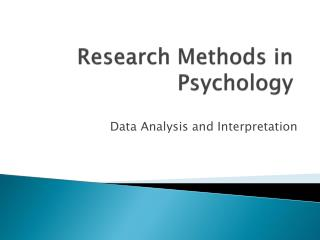Research Methods in Psychology

# Research Methods in Psychology - PowerPoint PPT Presentation

Research Methods in Psychology. Data Analysis and Interpretation. Null Hypothesis Significance Testing (NHST).I am the owner, or an agent authorized to act on behalf of the owner, of the copyrighted work described.
Download Presentation## Research Methods in Psychology

An Image/Link below is provided (as is) to download presentation

Download Policy: Content on the Website is provided to you AS IS for your information and personal use and may not be sold / licensed / shared on other websites without getting consent from its author.While downloading, if for some reason you are not able to download a presentation, the publisher may have deleted the file from their server.

- - - - - - - - - - - - - - - - - - - - - - - - - - E N D - - - - - - - - - - - - - - - - - - - - - - - - - -
Presentation Transcript### Research Methods in Psychology

Data Analysis and Interpretation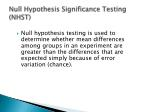Null Hypothesis Significance Testing (NHST)
• Null hypothesis testing is used to determine whether mean differences among groups in an experiment are greater than the differences that are expected simply because of error variation (chance).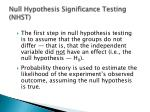Null Hypothesis Significance Testing (NHST)
• The first step in null hypothesis testing is to assume that the groups do not differ — that is, that the independent variable did not have an effect (i.e., the null hypothesis — H0).
• Probability theory is used to estimate the likelihood of the experiment’s observed outcome, assuming the null hypothesis is true.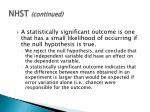NHST (continued)
• A statistically significant outcome is one that has a small likelihood of occurring if the null hypothesis is true.
• We reject the null hypothesis, and conclude that the independent variable did have an effect on the dependent variable.
• A statistically significant outcome indicates that the difference between means obtained in an experiment is larger than would be expected if error variation alone (i.e., chance) were responsible for the outcome.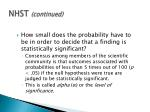NHST (continued)
• How small does the probability have to be in order to decide that a finding is statistically significant?
• Consensus among members of the scientific community is that outcomes associated with probabilities of less than 5 times out of 100 (p < .05) if the null hypothesis were true are judged to be statistically significant.
• This is called alpha (α) or the level of significance.NHST (continued)
• What does a statistically significant outcome tell us?
• An outcome with a probability just below .05 (and thus statistically significant) has about a 50/50 chance of being repeated in an exact replication of the experiment.
• As the probability of the outcome of the experiment decreases (e.g., p = .025, p = .01, p = .005), the likelihood of observing a statistically significant outcome (p< .05) in an exact replication increases.
• APA recommends reporting the exact probability of the outcome.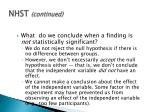NHST (continued)
• What do we conclude when a finding is not statistically significant?
• We do not reject the null hypothesis if there is no difference between groups.
• However, we don’t necessarily accept the null hypothesis either — that is, we don’t conclude that the independent variable did not have an effect.
• We cannot make a conclusion about the effect of the independent variable. Some factor in the experiment may have prevented us from observing an effect of the independent variable (e.g., too few participants).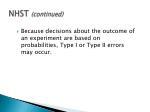NHST (continued)
• Because decisions about the outcome of an experiment are based on probabilities, Type I or Type II errors may occur.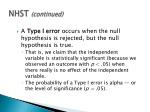NHST (continued)
• A Type I error occurs when the null hypothesis is rejected, but the null hypothesis is true.
• That is, we claim that the independent variable is statistically significant (because we observed an outcome with p< .05) when there really is no effect of the independent variable.
• The probability of a Type I error is alpha — or the level of significance (p = .05).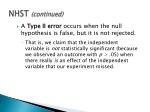NHST (continued)
• A Type II error occurs when the null hypothesis is false, but it is not rejected.
• That is, we claim that the independent variable is not statistically significant (because we observed an outcome with p > .05) when there really is an effect of the independent variable that our experiment missed.NHST (continued)
• Because of the possibility of Type I and Type II errors, researchers are tentative in their claims. We use words such as “support for the hypothesis” or “consistent with the hypothesis” rather than stating that a hypothesis has been “proven.”NHST: Comparing Two Means
• The appropriate inferential statistical test when comparing two means obtained from different groups of participants is a t -test for independent groups.
• The appropriate test when comparing two means obtained from the same participants (or matched groups) is a repeated measures (within-subjects) t-test.T-test for Paired Samples
• Research Design
• Analysis of Independent Variable using two conditions
• Experimental
• Control
• Same group of subjects is used
• Each subject receives the experimental and control
• Subjects may be also be matched according to certain characteristicsT-test for Paired Samples
• Statistic is based on the difference between the scores of correlated subjects
• Score compared to a difference of 0
• Null hypothesis assumes no difference
• Population mean is equal to 0
• T critical obtained in same manner as t test for single samplesANOVA
• Used to analyze data from experiments that use more than two groups or conditions
• F is a ratio of two independent variance estimates
• Since F is a ratio of variance estimates, it will never be negativeOverview of ANOVA technique
• F test allows us to make one overall comparison that tells whether there is a significant difference between the means of the groupsIntroduction F Distribution
• F distribution
• F distribution is positively skewed
• Median F value equals one
• F distribution is a family of curves based on the degrees of freedom (df)Overview of ANOVA technique
• In the independent groups design, there are as many groups as there are levels of the independent variable
• Hypothesis testing:
• Nondirectional
• H0 states that there is no difference between conditionsOverview of ANOVA technique
• ANOVA partitions total variability of data (SST) into the variability that exists within each group (SSW) and the variability between groups (SSB)
• SS= Sum of SquaresOverview of ANOVA technique
• SSB andSSW are both used as independent estimates of the H0 population variance
• F ratioANOVA (continued)
• The ANOVA Summary Table provides the information for estimating the sources of variance: between groups and within groups.

Source Sum of Squares (SS) df Variance Estimate F-test p

Between Groups 54.55 3 18.18 7.80 .002

Within Groups 37.20 16 2.33

• The F-test is the Between group variance estimate is divided by the within group variance estimate(18.18 ÷ 2.33 = 7.80).
• This F-test is statistically significant because .002 < .05.